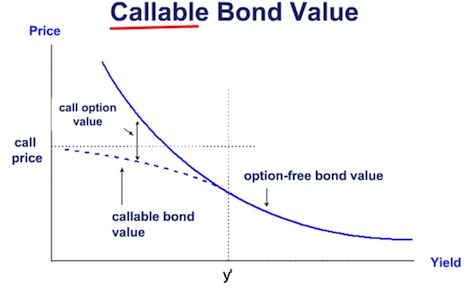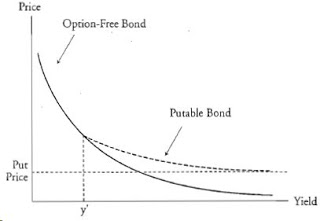#### Subject 3. Properties of Bond Duration

Bond duration is affected by many variables.

• The fraction of the period that has gone by (t/T). A plot of Macaulay duration (or modified duration) against time for a single bond with constant yield will show a saw-tooth pattern, with Macaulay duration declining steadily until a coupon payment results in an upwards jump.

• The Macaulay duration of a zero-coupon bond is its time-to-maturity.

• The Macaulay duration of a perpetual bond (perpetuity) is (1 + r) / r.

• Coupon rate is inversely related to Macaulay duration and modified duration.

• Yield-to-maturity is also inversely related to Macaulay duration and modified duration.

• Time-to-maturity and Macaulay and modified duration are usually positively related.

• They are always positively related on bonds priced at par or at a premium above par value.
• They are usually positively related on bonds priced at par or at a discount below par value. The exception is long-term, low coupon bonds, on which it is possible to have a lower duration than on an otherwise comparable shorter-term bond.

Callable Bonds

A callable bond exhibits positive convexity at high yield levels and negative convexity at low yield levels. Negative convexity means that for a large change in interest rates, the amount of the price appreciation is less than the amount of the price depreciation.• When the required yield for the callable bond is higher than its coupon rate, the bond is unlikely to be called. Therefore, the callable bond will have a similar price/yield relationship (positive convexity) as a comparable option-free bond.
• When the required yield becomes lower than the coupon rate, the value of the call option increases because it is getting more and more likely that the bond may be retired at the call price. The call price will set an upper limit on the price of the callable bond. In contrast, for an option-free bond, the bond price will rise unabated as the yield falls. If the required yield rises (but not higher than the coupon rate), the price of the non-callable bond falls and the price of the call option falls. As the price of a callable bond is the difference between the price of the non-callable bond and the price of the embedded option, the price of a callable bond will not fall as much as a non-callable bond. Therefore, a callable bond exhibits negative convexity at low yield levels.

Putable Bonds

The difference between the value of a putable bond and the value of an otherwise comparable option-free bond is the value of the embedded put option.• When the required yield for the putable bond is low relative to the issuer's coupon rate, the price of the putable bond is basically the same as the price of the option-free bond because the value of the put option is small. An investor will not sell the bond to the issuer at the put price. Therefore, the putable bond will have a similar price/yield relationship to a comparable option-free bond.
• As rates rise, the price of the putable bond declines, but the price decline is less than that for an option-free bond. The value of the put option increases because it's getting more and more likely that the investor will sell the bond to the issuer at the put price. Therefore, the put price sets a lower limit on the price of the putable bond.